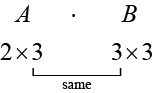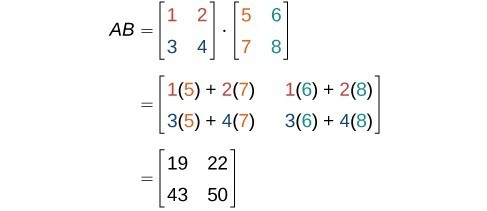## Finding the Product of Two Matrices

In addition to multiplying a matrix by a scalar, we can multiply two matrices. Finding the product of two matrices is only possible when the inner dimensions are the same, meaning that the number of columns of the first matrix is equal to the number of rows of the second matrix. If $A$ is an $\text{ }m\text{ }\times \text{ }r\text{ }$ matrix and $B$ is an $\text{ }r\text{ }\times \text{ }n\text{ }$ matrix, then the product matrix $AB$ is an $\text{ }m\text{ }\times \text{ }n\text{ }$ matrix. For example, the product $AB$ is possible because the number of columns in $A$ is the same as the number of rows in $B$. If the inner dimensions do not match, the product is not defined.Figure 1

We multiply entries of $A$ with entries of $B$ according to a specific pattern as outlined below. The process of matrix multiplication becomes clearer when working a problem with real numbers.

To obtain the entries in row $i$ of $AB,\text{}$ we multiply the entries in row $i$ of $A$ by column $j$ in $B$ and add. For example, given matrices $A$ and $B,\text{}$ where the dimensions of $A$ are $2\text{ }\times \text{ }3$ and the dimensions of $B$ are $3\text{ }\times \text{ }3,\text{}$ the product of $AB$ will be a $2\text{ }\times \text{ }3$ matrix.

$A=\left[\begin{array}{rrr}\hfill {a}_{11}& \hfill {a}_{12}& \hfill {a}_{13}\\ \hfill {a}_{21}& \hfill {a}_{22}& \hfill {a}_{23}\end{array}\right]\text{ and }B=\left[\begin{array}{rrr}\hfill {b}_{11}& \hfill {b}_{12}& \hfill {b}_{13}\\ \hfill {b}_{21}& \hfill {b}_{22}& \hfill {b}_{23}\\ \hfill {b}_{31}& \hfill {b}_{32}& \hfill {b}_{33}\end{array}\right]$

Multiply and add as follows to obtain the first entry of the product matrix $AB$.

1. To obtain the entry in row 1, column 1 of $AB,\text{}$ multiply the first row in $A$ by the first column in $B$, and add.
$\left[\begin{array}{ccc}{a}_{11}& {a}_{12}& {a}_{13}\end{array}\right]\cdot \left[\begin{array}{c}{b}_{11}\\ {b}_{21}\\ {b}_{31}\end{array}\right]={a}_{11}\cdot {b}_{11}+{a}_{12}\cdot {b}_{21}+{a}_{13}\cdot {b}_{31}$
2. To obtain the entry in row 1, column 2 of $AB,\text{}$ multiply the first row of $A$ by the second column in $B$, and add.
$\left[\begin{array}{ccc}{a}_{11}& {a}_{12}& {a}_{13}\end{array}\right]\cdot \left[\begin{array}{c}{b}_{12}\\ {b}_{22}\\ {b}_{32}\end{array}\right]={a}_{11}\cdot {b}_{12}+{a}_{12}\cdot {b}_{22}+{a}_{13}\cdot {b}_{32}$
3. To obtain the entry in row 1, column 3 of $AB,\text{}$ multiply the first row of $A$ by the third column in $B$, and add.
$\left[\begin{array}{ccc}{a}_{11}& {a}_{12}& {a}_{13}\end{array}\right]\cdot \left[\begin{array}{c}{b}_{13}\\ {b}_{23}\\ {b}_{33}\end{array}\right]={a}_{11}\cdot {b}_{13}+{a}_{12}\cdot {b}_{23}+{a}_{13}\cdot {b}_{33}$

We proceed the same way to obtain the second row of $AB$. In other words, row 2 of $A$ times column 1 of $B$; row 2 of $A$ times column 2 of $B$; row 2 of $A$ times column 3 of $B$. When complete, the product matrix will be

$AB=\left[\begin{array}{c}\begin{array}{l}{a}_{11}\cdot {b}_{11}+{a}_{12}\cdot {b}_{21}+{a}_{13}\cdot {b}_{31}\\ \end{array}\\ {a}_{21}\cdot {b}_{11}+{a}_{22}\cdot {b}_{21}+{a}_{23}\cdot {b}_{31}\end{array}\begin{array}{c}\begin{array}{l}{a}_{11}\cdot {b}_{12}+{a}_{12}\cdot {b}_{22}+{a}_{13}\cdot {b}_{32}\\ \end{array}\\ {a}_{21}\cdot {b}_{12}+{a}_{22}\cdot {b}_{22}+{a}_{23}\cdot {b}_{32}\end{array}\begin{array}{c}\begin{array}{l}{a}_{11}\cdot {b}_{13}+{a}_{12}\cdot {b}_{23}+{a}_{13}\cdot {b}_{33}\\ \end{array}\\ {a}_{21}\cdot {b}_{13}+{a}_{22}\cdot {b}_{23}+{a}_{23}\cdot {b}_{33}\end{array}\right]$

### A General Note: Properties of Matrix Multiplication

For the matrices $A,B,\text{}$ and $C$ the following properties hold.

• Matrix multiplication is associative: $\left(AB\right)C=A\left(BC\right)$.
• Matrix multiplication is distributive: $\begin{array}{l}\begin{array}{l}\\ C\left(A+B\right)=CA+CB,\end{array}\hfill \\ \left(A+B\right)C=AC+BC.\hfill \end{array}$

Note that matrix multiplication is not commutative.

### Example 8: Multiplying Two Matrices

Multiply matrix $A$ and matrix $B$.

$A=\left[\begin{array}{cc}1& 2\\ 3& 4\end{array}\right]\text{ and }B=\left[\begin{array}{cc}5& 6\\ 7& 8\end{array}\right]$

### Solution

First, we check the dimensions of the matrices. Matrix $A$ has dimensions $2\times 2$ and matrix $B$ has dimensions $2\times 2$. The inner dimensions are the same so we can perform the multiplication. The product will have the dimensions $2\times 2$.

We perform the operations outlined previously.Figure 2

### Example 9: Multiplying Two Matrices

Given $A$ and $B:$

1. Find $AB$.
2. Find $BA$.
$A=\left[\begin{array}{l}\begin{array}{ccc}-1& 2& 3\end{array}\hfill \\ \begin{array}{ccc}4& 0& 5\end{array}\hfill \end{array}\right]\text{ and }B=\left[\begin{array}{c}5\\ -4\\ 2\end{array}\begin{array}{c}-1\\ 0\\ 3\end{array}\right]$

### Solution

1. As the dimensions of $A$ are $2\text{}\times \text{}3$ and the dimensions of $B$ are $3\text{}\times \text{}2,\text{}$ these matrices can be multiplied together because the number of columns in $A$ matches the number of rows in $B$. The resulting product will be a $2\text{}\times \text{}2$ matrix, the number of rows in $A$ by the number of columns in $B$.
$\begin{array}{l}\hfill \\ AB=\left[\begin{array}{rrr}\hfill -1& \hfill 2& \hfill 3\\ \hfill 4& \hfill 0& \hfill 5\end{array}\right]\text{ }\left[\begin{array}{rr}\hfill 5& \hfill -1\\ \hfill -4& \hfill 0\\ \hfill 2& \hfill 3\end{array}\right]\hfill \\ \text{ }=\left[\begin{array}{rr}\hfill -1\left(5\right)+2\left(-4\right)+3\left(2\right)& \hfill -1\left(-1\right)+2\left(0\right)+3\left(3\right)\\ \hfill 4\left(5\right)+0\left(-4\right)+5\left(2\right)& \hfill 4\left(-1\right)+0\left(0\right)+5\left(3\right)\end{array}\right]\hfill \\ \text{ }=\left[\begin{array}{rr}\hfill -7& \hfill 10\\ \hfill 30& \hfill 11\end{array}\right]\hfill \end{array}$
2. The dimensions of $B$ are $3\times 2$ and the dimensions of $A$ are $2\times 3$. The inner dimensions match so the product is defined and will be a $3\times 3$ matrix.
$\begin{array}{l}\hfill \\ BA=\left[\begin{array}{rr}\hfill 5& \hfill -1\\ \hfill -4& \hfill 0\\ \hfill 2& \hfill 3\end{array}\right]\text{ }\left[\begin{array}{rrr}\hfill -1& \hfill 2& \hfill 3\\ \hfill 4& \hfill 0& \hfill 5\end{array}\right]\hfill \\ \text{ }=\left[\begin{array}{rrr}\hfill 5\left(-1\right)+-1\left(4\right)& \hfill 5\left(2\right)+-1\left(0\right)& \hfill 5\left(3\right)+-1\left(5\right)\\ \hfill -4\left(-1\right)+0\left(4\right)& \hfill -4\left(2\right)+0\left(0\right)& \hfill -4\left(3\right)+0\left(5\right)\\ \hfill 2\left(-1\right)+3\left(4\right)& \hfill 2\left(2\right)+3\left(0\right)& \hfill 2\left(3\right)+3\left(5\right)\end{array}\right]\hfill \\ \text{ }=\left[\begin{array}{rrr}\hfill -9& \hfill 10& \hfill 10\\ \hfill 4& \hfill -8& \hfill -12\\ \hfill 10& \hfill 4& \hfill 21\end{array}\right]\hfill \end{array}$

### Analysis of the Solution

Notice that the products $AB$ and $BA$ are not equal.

$AB=\left[\begin{array}{cc}-7& 10\\ 30& 11\end{array}\right]\ne \left[\begin{array}{ccc}-9& 10& 10\\ 4& -8& -12\\ 10& 4& 21\end{array}\right]=BA$

This illustrates the fact that matrix multiplication is not commutative.

### Is it possible for AB to be defined but not BA?

Yes, consider a matrix A with dimension $3\times 4$ and matrix B with dimension $4\times 2$. For the product AB the inner dimensions are 4 and the product is defined, but for the product BA the inner dimensions are 2 and 3 so the product is undefined.

### Example 10: Using Matrices in Real-World Problems

Let’s return to the problem presented at the opening of this section. We have the table below, representing the equipment needs of two soccer teams.

Wildcats Mud Cats
Goals 6 10
Balls 30 24
Jerseys 14 20

We are also given the prices of the equipment, as shown in the table below.

 Goal $300 Ball$10 Jersey $30 We will convert the data to matrices. Thus, the equipment need matrix is written as $E=\left[\begin{array}{c}6\\ 30\\ 14\end{array}\begin{array}{c}10\\ 24\\ 20\end{array}\right]$ The cost matrix is written as $C=\left[\begin{array}{ccc}300& 10& 30\end{array}\right]$ We perform matrix multiplication to obtain costs for the equipment. $\begin{array}{l}\hfill \\ \hfill \\ CE=\left[\begin{array}{rrr}\hfill 300& \hfill 10& \hfill 30\end{array}\right]\cdot \left[\begin{array}{rr}\hfill 6& \hfill 10\\ \hfill 30& \hfill 24\\ \hfill 14& \hfill 20\end{array}\right]\hfill \\ \text{ }=\left[\begin{array}{rr}\hfill 300\left(6\right)+10\left(30\right)+30\left(14\right)& \hfill 300\left(10\right)+10\left(24\right)+30\left(20\right)\end{array}\right]\hfill \\ \text{ }=\left[\begin{array}{rr}\hfill 2,520& \hfill 3,840\end{array}\right]\hfill \end{array}$ The total cost for equipment for the Wildcats is$2,520, and the total cost for equipment for the Mud Cats is \$3,840.

### How To: Given a matrix operation, evaluate using a calculator.

1. Save each matrix as a matrix variable $\left[A\right],\left[B\right],\left[C\right],..$.
2. Enter the operation into the calculator, calling up each matrix variable as needed.
3. If the operation is defined, the calculator will present the solution matrix; if the operation is undefined, it will display an error message.

### Example 11: Using a Calculator to Perform Matrix Operations

Find $AB-C$ given

$A=\left[\begin{array}{rrr}\hfill -15& \hfill 25& \hfill 32\\ \hfill 41& \hfill -7& \hfill -28\\ \hfill 10& \hfill 34& \hfill -2\end{array}\right],B=\left[\begin{array}{rrr}\hfill 45& \hfill 21& \hfill -37\\ \hfill -24& \hfill 52& \hfill 19\\ \hfill 6& \hfill -48& \hfill -31\end{array}\right],\text{and }C=\left[\begin{array}{rrr}\hfill -100& \hfill -89& \hfill -98\\ \hfill 25& \hfill -56& \hfill 74\\ \hfill -67& \hfill 42& \hfill -75\end{array}\right]$.

### Solution

On the matrix page of the calculator, we enter matrix $A$ above as the matrix variable $\left[A\right]$, matrix $B$ above as the matrix variable $\left[B\right]$, and matrix $C$ above as the matrix variable $\left[C\right]$.

On the home screen of the calculator, we type in the problem and call up each matrix variable as needed.

$\left[A\right]\times \left[B\right]-\left[C\right]$

The calculator gives us the following matrix.

$\left[\begin{array}{rrr}\hfill -983& \hfill -462& \hfill 136\\ \hfill 1,820& \hfill 1,897& \hfill -856\\ \hfill -311& \hfill 2,032& \hfill 413\end{array}\right]$